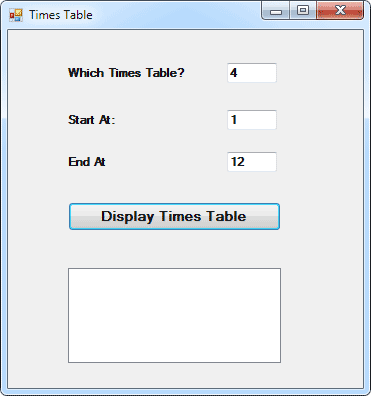# Arrays where the Boundaries are not known

This lesson is part of an ongoing tutorial. This first part is here: What is an Array?

Study the following Form:In the above Form, the user is invited to enter values into three Textboxes. The first Textbox is for whatever times table he or she wants. The second Textbox asks for the starting value of the times table. The third Textbox is for the end number of the times table. In other words, 1 times 4, 2 times 4, 3 times 4, right up to 12 times 4.

The point is that the user can enter any values he or she wants. We won't know until they are entered, and the button is clicked. Up until now, we've used an array with a fixed size. Our previous times table programme only went up to 10, and it started at 1. We used this to set up our array:

Dim numbers(10) As Integer

But that array would be no good for the above Form. Our array only held 11 positions. The user definitely wants the 4 times table right up to 12. Is there any way we can set up an array where the number of positions is not known? How can we set up a Non-Fixed size array?

You do it like this. First set up an array with empty brackets

Dim numbers( ) As Integer

Next, pass the values from the Text Boxes to some variables

times = Val(Textbox1.Text)
startAt = Val(Textbox2.Text)
endAt = Val(Textbox3.Text)

We can then use these values to reset the array. You reset an array by using the ReDim word. You then specify the new values. Like this:

ReDim numbers(endAt)

Our original array did not have its size set - Dim numbers( ) As Integer. So we've got the end number from the Textbox. When we reset an array, we can use this new value. Since our user entered the value 12 for the end number, our array is now really this:

Dim numbers(12) As Integer

We can use the same variables for our For Loop. Then we can go round and round the loop assigning values to our array.

No more reading these lessons online - get the eBook here!

To test this concept out, either start a new project, or amend the one you have displayed. Create three textboxes and labels. And add a new Button. Double click your button to open the code window. Then add the following code (the important line is in red):

Dim numbers() As Integer
Dim startAt As Integer
Dim endAt As Integer
Dim times As Integer
Dim i As Integer

times = Val(TextBox1.Text)
startAt = Val(TextBox2.Text)
endAt = Val(TextBox3.Text)

ReDim numbers(endAt)

For i = startAt To endAt